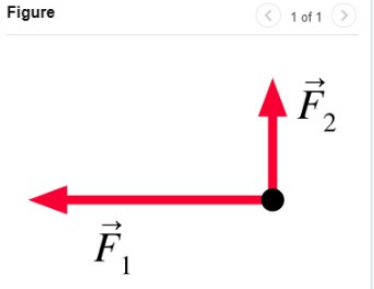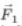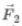# Problem: Two forces act on an object at rest as shown in (Figure 1)Add a third force that results in a net force of zero.Draw the vector starting at the black dot. The location, orientation, and length of the vector will be graded. You can move the vectors  and  to construct the required vector.

###### FREE Expert Solution

The third force should be in a direction that opposes both F1 and F2.

86% (495 ratings)###### Problem Details

Two forces act on an object at rest as shown in (Figure 1)Add a third force that results in a net force of zero.

Draw the vector starting at the black dot. The location, orientation, and length of the vector will be graded. You can move the vectorsandto construct the required vector.

Frequently Asked Questions

What scientific concept do you need to know in order to solve this problem?

Our tutors have indicated that to solve this problem you will need to apply the Equilibrium in 2D concept. You can view video lessons to learn Equilibrium in 2D. Or if you need more Equilibrium in 2D practice, you can also practice Equilibrium in 2D practice problems.

What professor is this problem relevant for?

Based on our data, we think this problem is relevant for Professor Dominguez's class at MDC.